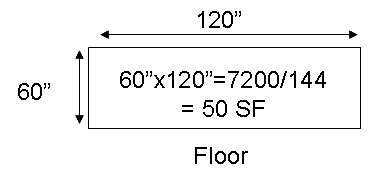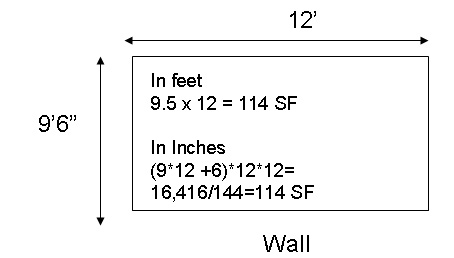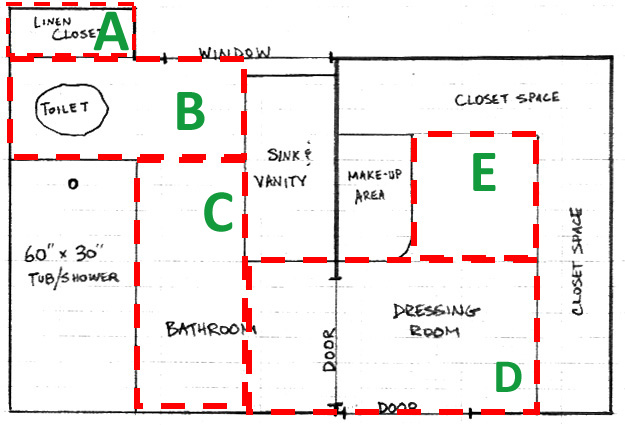# How to Calculate Your Tile Requirements

 Materials Sold by the Square Foot Calculating your material needs when you are purchasing by the square foot requires that you determine the areas you wish to cover and sum the total square feet of each of those areas.You can calculate the quantity in inches and divide by 144 to obtain the quantity in square feet.You can convert the measurements in feet to inches. The total square footage of these areas for both the floor and wall is 164 Square feet. (114+60=164) Keep in mind that you will most likely need to order a little bit extra of materials than what you need to cover your actual square footage. Usually you will order about 5-10% extra, but this can vary depending on several things, some, but not all are: 1) The layout of your area (a simple straight rectangle will require less additional material than a room with many angles). Rule of thumb 10% for straight spaces and 15% for irregular spaces. 2) The type of flooring you choose. Mosaics which are mesh mounted, can easily be cut and then inserted into angles or other void areas, while tiles which have been cut may not precisely fit into those types of spaces. 3) The direction you lay your tile (if you choose tile as your flooring). The rule of thumb is 10% for a straight layout and 15% for a diagonal layout. 4) How much extra material you want to have left over after your job is complete. In reality, bathroom spaces look more like this drawing. You can do your own hand drawing of the room and then divide up the drawing into the areas which will be tiled.Now you will need to calculate the length and width of areas A through E and total them. You may also create rectangles around areas which you wish to deduct form your total.